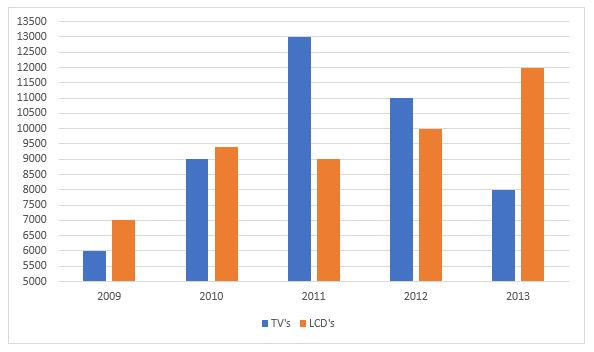# Data Interpretation Questions for SSC CGL

0
2861

Data Interpretation Questions for SSC CGL:

Download Data Interpretation questions with answers for SSC CGL exam, Provided with detailed explanations and solutions. Solve very important DI Problems for SSC CGL preparation. Download Data Interpretation questions PDF.

Instructions:

Study the following bar diagram carefully and answer the following questions.

The number of the production of electronic items (TVs and LCDs) in a factory during the period from 2009 to 2013.Question 1:

The total number of products of electronic items is maximum in the year

a) 2009
b) 2010
c) 2011
d) 2013

Question 2:

The ratio of production of LCDs in the year 2011 and 2013 is

a) 3 : 4
b) 4 : 3
c) 2 : 3
d) 1 : 4

Question 3:

The difference between averages of production of TVs and LCDs from 2009 to 2012 is

a) 600
b) 700
c) 800
d) 900

Question 4:

The ratio of production of TVs in the years 2009 and 2010 is

a) 7 : 6
b) 6 : 7
c) 2 : 3
d) 3 : 2

Solutions 1 to 5

Total no. of electronic items in :
2009 = 6000 + 7000 = 13000
2010 = 9000 + 9400 = 18400
2011 = 13000 + 9000 = 22000 [MAX]
2012 = 11000 + 10000 = 21000
2013 = 8000 + 12000 = 20000
=> Total production is maximum in 2011

Total production of LCD’s in 2011 = 9000
Total production of LCD’s in 2013 = 12000
Require ratio = 9000/12000 = 3 : 4